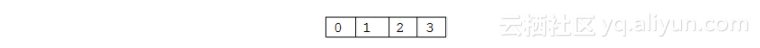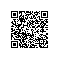# 《C和C++代码精粹》——2.10　指针和多维数组

### 2.10　指针和多维数组

C和C++代码精粹

int  a={0,1,2,3};int a={{0,1,2,3},{4,5,6,7},{8,9,0,1}}int a[]={0,1,2,3},*p=a;
/*...*/
p[i]=...


int a[]={ {0,2,3,4},{4,5,6,7},{8,9,0,1}};
int **p=a;      /*受怀疑的指针转换*/
/*...*/
p[i][j]=...


*(p[i]+j)


*(*(p+i)+j)


// array8.cpp:    使用一个一维数组指针
#include <iostream>

main()
{
int a[] = {{0,1,2,3},{4,5,6,7},{8,9,0,1}};
int (*p) = a;        //指向包含4个整型成员的数组
size_t nrows = sizeof a / sizeof a;
size_t ncols = sizeof a / sizeof a;

cout << "sizeof(*p) == " << sizeof *p << endl;
for (int i = 0; i < nrows; ++i)
{
for (int j = 0; j < ncols; ++j)
cout << p[i][j] << ' ';
cout << endl;
}
}

//输出:
sizeof(*p) == 16
0 1 2 3
4 5 6 7
8 9 0 1


int (*p)=a;


1．p+i

2．*（p+i）

3．由于数组 *（p+i）不是 sizeof 或者 & 运算的操作数，所以它被指向它第一个元素的指针所替代，即 &ai ，这是一个指向整型（int）的指针。

4．&ai+j

5．*&ai

a[i]==a+i  //  错误！使用钉钉扫一扫加入圈子
+ 订阅